TopAsiaFX

5 Oct, 2023

# Trading Scenario: Margin Call Level at 100% and Stop Out Level at 50%

In this scenario, with a Margin Call Level at 100% and a Stop Out Level at 50%, when a trader's equity drops to or below 50% of their used margin, the broker forcefully closes positions to limit losses, as the margin call couldn't be met.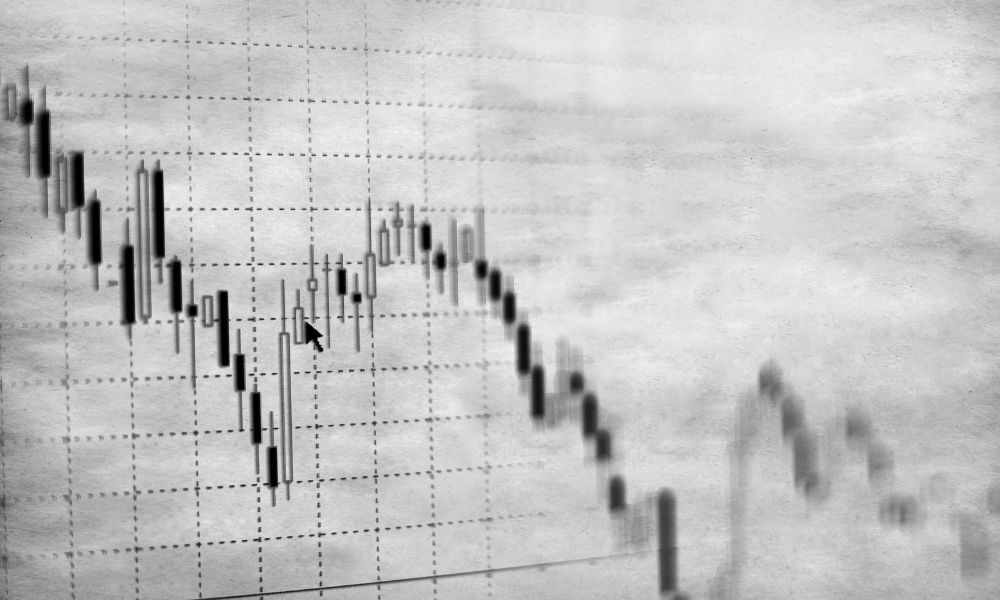Different retail forex brokers and CFD providers have varying margin call policies. Some exclusively employ Margin Calls, while others establish distinct Margin Call and Stop Out Levels.

In the previous lesson, we navigated through a trading scenario involving a broker that relied solely on Margin Calls.

In this lesson, we'll delve into a real-world trading scenario with a broker operating using separate Margin Call and Stop Out Levels.

The broker has designated the Margin Call Level at 100% and the Stop Out Level at 50%.

Let's explore what transpires in your margin account when confronted with a trade gone awry.

## Step 1: Deposit Funds into Trading Account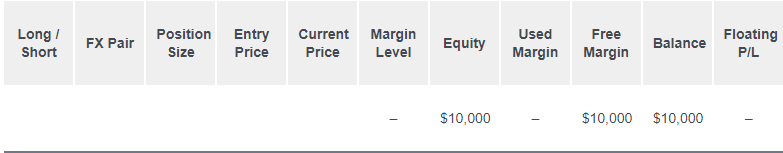## Step 2: Calculate Required Margin

Suppose you intend to go long on GBP/USD at 1.30000, opening a position of 1 standard lot (100,000 units). The Margin Requirement stands at 5%.

What amount of margin (Required Margin) must you allocate for this trade?

Given that your trading account is denominated in USD, it's essential to convert the GBP value into USD to ascertain the Notional Value of the trade.

• £1 = \$1.30

• £100,000 = \$130,000

Hence, the Notional Value equals \$130,000.

Now, we can compute the Required Margin:

• Required Margin = Notional Value x Margin Requirement

• \$6,500 = \$130,000 x .05

Assuming your trading account uses USD as its denomination and given the Margin Requirement of 5%, the Required Margin amounts to \$6,500.

## Step 3: Calculate Used Margin

With the exception of the trade you've just initiated, no other trades are currently active. Consequently, since you have only one position open, the Used Margin aligns with the Required Margin.

## Step 4: Calculate Equity

Let's assume that the market has marginally moved in your favor, with your position now trading at the break-even point. This signifies that your Floating P/L remains at \$0.

• Equity = Balance + Floating Profits (or Losses)
• \$10,000 = \$10,000 + \$0

Your account's Equity now stands at \$10,000.

## Step 5: Calculate Free Margin

With knowledge of your Equity, you can proceed to calculate the Free Margin:

• Free Margin = Equity - Used Margin

• \$3,500 = \$10,000 - \$6,500

Hence, your Free Margin is \$3,500.

## Step 6: Calculate Margin Level

Having determined your Equity, it's now possible to calculate the Margin Level:

• Margin Level = (Equity / Used Margin) x 100%

• 154% = (\$10,000 / \$6,500) x 100%

Your Margin Level registers at 154%.## GBP/USD drops 400 pips!

GBP/USD has declined by 400 pips and is currently trading at 1.26000. Let's assess the impact on your account.

### Used Margin

You'll observe that the Used Margin has undergone a change. Alterations in the exchange rate necessitate a recalculation of the Required Margin. Whenever there's a fluctuation in the GBP/USD price, the Required Margin adjusts accordingly.

With GBP/USD now trading at 1.26000 (as opposed to 1.30000), let's determine the Required Margin necessary to keep the position open, given that your trading account is in USD denomination.

• £1 = \$1.26

• £100,000 = \$126,000

Consequently, the Notional Value is \$126,000.

Previously, the Notional Value stood at \$130,000. The decline in GBP/USD signifies a weakening of the GBP. As your account is USD-denominated, this reduction in the Notional Value ensues.

We can now calculate the Required Margin:

• Required Margin = Notional Value x Margin Requirement

• \$6,300 = \$126,000 x .05

It's noteworthy that the decrease in Notional Value has led to a reduction in the Required Margin. With a Margin Requirement of 5%, the Required Margin equals \$6,300. In contrast, it was previously \$6,500 when GBP/USD traded at 1.30000. The adjustment in Required Margin applies to all open positions. In this instance, with only one position open, the Used Margin aligns with the new Required Margin.

### Floating P/L

GBP/USD's decline from 1.30000 to 1.26000, a variance of 400 pips, translates to a Floating Loss of \$4,000. Given that you're trading 1 standard lot, each pip movement amounts to \$10.

• Floating P/L = (Current Price - Entry Price) x 10,000 x \$X/pip

• -\$4,000 = (1.26000 - 1.30000) x 10,000 x \$10/pip

### Equity

Your Equity now stands at \$6,000.

• Equity = Balance + Floating P/L

• \$6,000 = \$10,000 + (-\$4,000)

### Free Margin

Your Free Margin has contracted to -\$300.

• Free Margin = Equity - Used Margin

• -\$300 = \$6,000 - \$6,300

### Margin Level

The Margin Level has dwindled to 95%.

• Margin Level = (Equity / Used Margin) x 100%

• 95% = (\$6,000 / \$6,300) x 100%

It's essential to note that the Margin Call Level is triggered when the Margin Level reaches 100%. Given your current Margin Level, a Margin Call is now imminent. It serves as a WARNING that your trade is at risk of being automatically closed. While your trade remains open, you'll be unable to initiate new positions until the Margin Level surpasses 100%.

### Account Metrics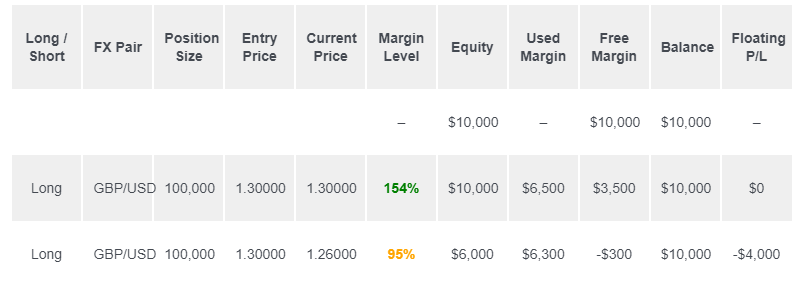## GBP/USD drops another 290 pips!

GBP/USD has declined by an additional 290 pips and is currently trading at 1.23100. Let's evaluate the impact on your account.

### Used Margin

The Used Margin has undergone a change as a result of the shift in exchange rates. Adjustments in the Required Margin are necessary whenever there's a fluctuation in GBP/USD. Given that GBP/USD is now trading at 1.23100 (in contrast to 1.26000), let's determine the Required Margin needed to maintain the open position.

• £1 = \$1.23100

• £100,000 = \$123,100

This yields a Notional Value of \$123,100. The Required Margin can now be calculated as follows:

• Required Margin = Notional Value x Margin Requirement

• \$6,155 = \$123,100 x .05

It's worth noting that the Required Margin has decreased in line with the reduction in Notional Value. Given a Margin Requirement of 5%, the Required Margin now stands at \$6,155, whereas it was previously \$6,300 when GBP/USD traded at 1.26000. In this example, where only one position is open, the Used Margin aligns with the new Required Margin.

### Floating P/L

The decline in GBP/USD from 1.30000 to 1.23100, spanning 690 pips, results in a Floating Loss of \$6,900. With 1 standard lot being traded, each pip's movement equates to \$10.

• Floating P/L = (Current Price - Entry Price) x 10,000 x \$X/pip

• -\$6,900 = (1.23100 - 1.30000) x 10,000 x \$10/pip

### Equity

Your Equity currently amounts to \$3,100.

• Equity = Balance + Floating P/L

• \$3,100 = \$10,000 + (-\$6,900)

### Free Margin

Your Free Margin has now contracted to -\$3,055.

• Free Margin = Equity - Used Margin

• -\$3,055 = \$3,100 - \$6,155

### Margin Level

The Margin Level has dwindled to 50%.

• Margin Level = (Equity / Used Margin) x 100%

• 50% = (\$3,100 / \$6,155) x 100%

At this juncture, your Margin Level has dipped below the Stop Out Level!

### Account Metrics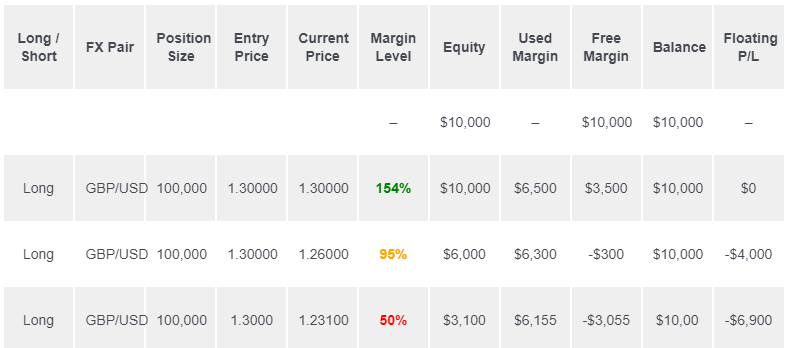## STOP OUT!

The Stop Out Level is triggered when the Margin Level descends to 50%.

At this juncture, your Margin Level has indeed reached the Stop Out Level!

Here's what transpires:

• Your Used Margin is released.

• Your Floating Loss becomes realized.

With your account now devoid of active positions and in a "flat" state, your Free Margin, Equity, and Balance assume identical values. As there are no open positions, the display lacks a Margin Level or Floating P/L.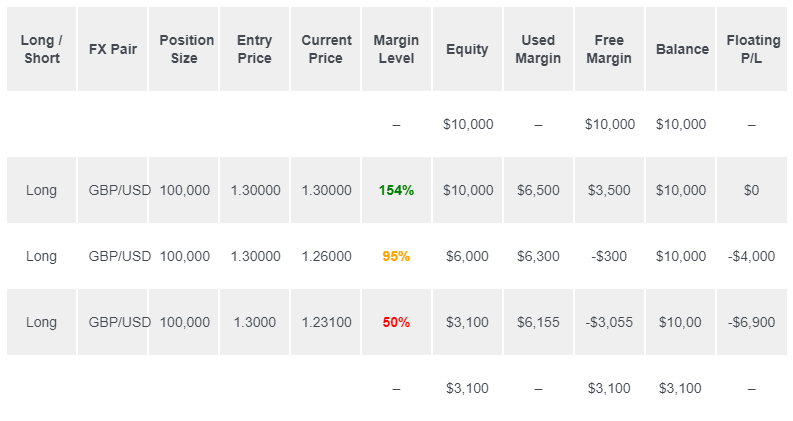Preceding the trade, you possessed \$10,000 in cash. However, you now find yourself with \$3,100!

This equates to a loss of 69% of your initial capital.

• % Gain/Loss = ((Ending Balance - Starting Balance) / Starting Balance) x 100%

• -69% = ((\$3,100 - \$10,000) / \$10,000) x 100%

It's worth noting that some traders experience a distressing reaction when they discover that their trade has been automatically liquidated.

In the forthcoming lesson, we'll present a distinct trading scenario in which you attempt to engage in forex trading with a mere \$100.

### Get news on social media!

Read our latest news on any of these social networks!